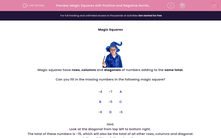# Complete Magic Squares with Positive and Negative Numbers

In this worksheet, students will solve problems with positive and negative numbers using 'Magic Squares'.Key stage:  KS 2

Curriculum topic:   Maths and Numerical Reasoning

Curriculum subtopic:   Square Numbers

Difficulty level:#### Worksheet Overview

Magic SquaresMagic squares have rows, columns and diagonals of numbers adding to the same total.

Can you fill in the missing numbers in the following magic square?

 −4 −7 A B −5 C −6 D −6

Hint:

Look at the diagonal from top left to bottom right.

The total of these numbers is -15, which will also be the total of all other rows, columns and diagonal.

Let's work through these together:

A = −4

-7 + -4 = -11

-11 + -4 = -15

= −3

-6 + -6 = -12

-15 - -12 = -3

= −5

We have already worked out that A = -4.

-6 + -4 = -10

-15 - -10 = -5

B = −5

We have already worked out that C = -5.

-5 + -5 = -10

-15 - -15 = -5

Now, it's over to you!

### What is EdPlace?

We're your National Curriculum aligned online education content provider helping each child succeed in English, maths and science from year 1 to GCSE. With an EdPlace account you’ll be able to track and measure progress, helping each child achieve their best. We build confidence and attainment by personalising each child’s learning at a level that suits them.

Get started